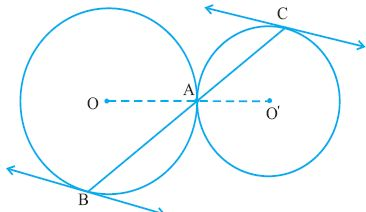Email us to get an instant 20% discount on highly effective K-12 Math & English kwizNET Programs!

#### Online Quiz (WorksheetABCD)

Questions Per Quiz = 2 4 6 8 10

### High School Mathematics - 28.13 Secants

 Theorem: (Tangent- Secant theorem or Alternate Segment theorem) The angle between chord AB and tangent PQ through the point of contact is equal to the angle in the alternate segment. Given: PQ is a tangent at A to the circle with O as center. AB is a chord. To prove: If C is a point on a major arc and D is a point on a minor arc with respect to the chord AB then BAQ = ACB and PAB = ADB Construction: Join OBDirections: Solve the following
 Q 1: Find the locus of the centre of circle of constant radius r, which touches a given circle of radius r1 internally.Answer: Q 2: Two rays ABP and ACQ are intersected by two parallel lines in B, C, P and Q respectively.Prove that the circumcircles of triangles ABC and APQ touch each other at A.Answer: Q 3: Two circles touch each other at points A and B. At A, tangents AP and AQ are drawn which intersect other circles at the points P and Q respectively. Prove that AB is the bisector of angle PBQ.Answer: Q 4: A circle with centre O intersects another circle with centre Ol in A and A and B passes through O. Tangent CD is drawn to the circle with sentre Ol. prove that OA is the bisector of angle CAB.Answer: Q 5: Find the locus of the centre of circle of constant radius r, which touches a given circle of radius r1 externally.Answer: Q 6: The diagonals of a parallelogram ABCD intersect at a point E. Show that the circumcircles of triangle ABE and BCE intersect at E.Answer: Q 7: Two circles with centres O and Ol touch internally at a point P. A line is drawn to pass through P intersecting the two circles at Q and R respectively. Prove that OQ ll OlR.Answer: Q 8: Two circles with centres O and Ol touch externally at point A . A line is drawn to intersect these circles at B and C. Prove that the tangents at B and C are parallel.Answer: Question 9: This question is available to subscribers only! Question 10: This question is available to subscribers only!

#### Subscription to kwizNET Learning System offers the following benefits:

• Unrestricted access to grade appropriate lessons, quizzes, & printable worksheets
• Instant scoring of online quizzes
• Progress tracking and award certificates to keep your student motivated
• Unlimited practice with auto-generated 'WIZ MATH' quizzes